# Analysis of Aspect Ratio Effects of Left Heated 2D Cavity Using Energy Streamlines and Field Synergy Principle

Analysis of Aspect Ratio Effects of Left Heated 2D Cavity Using Energy Streamlines and Field Synergy Principle

Hari Ponnamma Rani* Vekamulla Narayana  Yadagiri Rameshwar

Department of Mathematics, National Institute of Technology, Warangal 506004, India

Department of Mathematics, College of Engineering, Osmania University, Hyderabad 500007, India

Corresponding Author Email:
hprani@nitw.ac.in
Page:
437-448
|
DOI:
https://doi.org/10.18280/mmep.060316
17 November 2018
|
Accepted:
4 February 2019
|
Published:
15 September 2019
| Citation

OPEN ACCESS

Abstract:

In the present study natural convective flow of air in a two dimensional cavity with three different aspect ratios (AR) are analyzed using direct numerical simulation. Established open source CFD package OpenFOAM, has been utilized to run the simulation. In this problem, the vertical walls are assumed to be maintained at an isothermal temperature with left vertical wall has high temperature than the right wall and the other walls are assumed to be thermally insulated. The governing equations of this problem are expressed in dimensionless form and are solved by using the finite volume method. For spatial derivatives, the second order upwind linearization technique was used. The CGS method was employed as an effective acceleration means. The divergent and Laplacian terms in the governing equations are discretized by the QUICK and Gauss linear schemes, respectively. The computations are conducted for Rayleigh number until 106. An attempt has been made to gather the visualization techniques such as streamlines, isotherms, energy streamlines and field synergy principle to analyse the flow behavior. When Ra is small, vertical energy streamlines appeared, as Ra further increases, free energy streamlines at the boundary are observed whereas trapped energy streamlines at the centre appeared in the horizontal direction. For a fixed Ra, as AR increases, average synergy angle $\left(\beta_{m}\right)$ increases. This result implies that synergy between temperature field and velocity gets worse, which leads to the mild growth rate of $\overline{N u}$ The field synergy principle shows that the improving synergy between the temperature gradient and velocity vector, the heat transfer can be enhanced with less increased flow resistance.

Keywords:

Rayliegh number, aspect ratio, energy streamlines, field synergy, Nusselt number

1. Introduction

Laminar natural convection in closed enclosures was broadly studied both experimentally and numerically on account of special interest in large number of engineering applications: nuclear reactors, solar collectors, refrigeration in electronic components. In the past few decades, many experimental and theoretical studies on laminar natural convection in closed enclosures are studied. Because of this, the heat and fluid flow caused by laminar natural convection has received substantial awareness from numerous researchers. Recent work of Altac and Ugurlubilek  shows that research is continuing on these issues, with regard to three-dimensional effect and various turbulence models. In this line another recent study carried out by Obyn and Van Moeseke  with the focus on the impact of convective heat transfer coefficient. They used their computations to calculate the heating and cooling loads of buildings.

Fluids, such as air, in the absence of external forces like fans or exterior wind, move due to density variations in their bulk. These density variations are caused by temperature gradients and buoyancy forces that appear in the presence of gravity. Consequently, the rising of low-density particles occurs along with the falling of high-density particles; this phenomenon is known as natural or free convection. Inside buildings where free and forced convection coexist, the common practice is to evaluate the importance of each convection type separately, to determine whether either is dominant with respect to the other or if they must be considered simultaneously .

In thermal simulation programs, such as ESP-r, Energy Plus , DOE-2, and TRNSYS [4-7], the CHTC is fixed as a constant value; or, at most, the programs make the coefficients depend on the velocity and temperature difference between the surfaces. In such cases, a flat-plate correlation is used, or another empirical correlation is applied as obtained from Awbi and Hatton , or Novoselac et al. . On the other hand in several cases, the flow pattern, which is one of the salient factors in calculating such heat transfer coefficients, is not taken into account. This flow pattern directly relies on the problem and its geometry, such as the enclosure aspect ratio (AR).

From the literature survey [10-12], it is found that plotting of velocity vectors, streamlines and isotherms are the general visualization tools. The other visualization technique using the theory of heatfunction and heatlines was proposed by Kimura and Bejan . In the given domain for the visualization of energy flow, Heatline concept was used. Later Costa [14, 15] presented an approach to visualize the physical aspects of the flow with the aid of the heatfunction and heatlines. Similar to heatlines Mahmud and Fraser  found new visualization technique related to the convective heat transfer, called, energy streamlines. Hooman  introduced and developed new visualization tool known as the energy flux vectors. These vectors represent the flow of energy and are tangent to heatlines [18, 19].

Along with the idea of field synergy, interlink between the conduction and convection states was suggested by Guo et al. [20, 21]. The angle between the speed and isotherms is known as the synergy angle. However, they observed in the absence of fluid movement, only for diffusive heat transfer, the heat flux is parallel to both temperature gradient and velocity. Thus, the heatlines are vertical to the isotherms. But to get a convective heat transfer without a diffusive flux, the heat flux must be parallel to the velocity vector.

Mallinson and de Vahl Davis  performed calculations until Ra ~ 106 for the 3D geometry. Later Pepper and Hollands  studied flow in the 3D enclosures and paid attention on a range of Ra varying from laminar to turbulence. The AR effects with 1 ≤ AR ≤ 2 for 106 Ra ≤ 108 in the 3D differentially heated cubical cavity was investigated by Ravnik et al.  using the boundary element method. According to results reported by Rincon Casado et al.  for AR= 1 three-dimensional effects are less pronounced with a reduction of 2–4% compared to a 2D model for Ra ≤ 1012. This is due to the fact that the 3D effect of the boundary layer is not significant in the average Nusselt number with high Ra. For this reason, the present work considers negligible 3D effects, which produces a saving in computational cost and a focus on the influence of the enclosure aspect ratio.

1.1 Previous experimental works on vertical surfaces

In the past, advanced experimental techniques were used, although not without difficulty; for the low absolute velocity values accurate experimental work was limited and whereas for a rectangular cavity, the fluid flow and heat transfer are highly sensitive to the experimental setup and boundary conditions. The majority of the most rigorous research in this field is oriented towards the study of rectangular enclosures, where heat flux is unidirectional; i.e., flotation is induced from the vertical walls or from the floor to the roof. This is reported in the general review works by Catton  and Ostrach  on the natural convection in closed cavities.

A considerable number of experimental works have been done in the past decade to comprehend turbulent flow in enclosures, heated and cooled from opposing isothermal faces. The increase in the Rayleigh number has been gradual, beginning with Ra =104 in previous years, to Ra ≥1010 in the recent decade. These experiments are need to provide benchmark data for other studies, such as that developed by Leong et al. , who reported the Nusselt number result for vertical walls for a cubical air-filled cavity in laminar flow (Ra= 4×104), tilted at 0°, 45°, or 90°. Betts and Bokhari  studied a tall enclosure in laminar natural convection for Ra =0.86×106and Ra =1.43×106. Mamun et al.  published an extension to previous work in which the mean Nusselt number for 104<Ra< 3×108 was calculated. The outcomes are viewed as reasonable for the testing of computational codes. Ampofo  conducted an experiment and collected benchmark data study of low level turbulent natural convection in an air filled vertical square cavity. Bairi  experimentally and numerically studied the natural convection in air-filled 2D tilted square cavities. In his study, different geometrical and thermal configurations were considered for 10 ≤ Ra ≤ 1010 and tilt angles ranging from 0 to 360. Saury et al.  presented an experimental work for large Ra numbers in a 4m high cavity with a horizontal cross-section equal to 0.86×1.00 m2 (4×1010 Ra ≤ 1.2 ×1011). Inan et al.  numerically and experimentally studied the heat transfer in a rectangular cavity. They simulated a double-skin façade and included natural convection with 8.59×109Ra≤ 1.41×1010, and a correlation for the Nusselt number was developed. However, in the past studies the AR analysis was paid very less attention due to difficulties in experimentation; therefore, the correlations from the experimental work are not general and the applicability is limited.

1.2 Previous numerical works on vertical surfaces

In numerical and theoretical studies, strong coupling of the boundary layer and the core flow makes the computation very difficult. In addition, the direct simulation of turbulent natural convection in a cavity is still too costly. Numerical results from various k-ε models are non-unique. In addition, none of the turbulence models can correctly predict whole velocity and temperature fields. The limitations of computer technologies have restricted the numerical studies to 2D models until the last few decades. Among the works that have studied heat transfer on vertical walls of a square enclosure with an adiabatic floor and ceiling, it is worth highlighting the developments by Barakos et al. , Markatos and Pericleous , De Vahl Davis and Jones , and Lari et al. , who studied the effects of radioactive transfer in participating media. These results are often used to validate methodologies and calculation models. More recently, Altac and Ugurlubilek  used the results reported by other authors for comparison with various models of turbulence. However, one can observe in these works that the influence of the AR on rectangular enclosures has been poorly studied. Bejan  provided further insight into the AR influence, although for small aspect ratios and only in the laminar regime. Osman et al.  continued this work, but the turbulent regime was not investigated. Trias et al.  developed a set of direct numerical simulations of a differentially heated cavity of AR 4 with adiabatic horizontal walls in turbulent flow. Five configurations based on the cavity height were presented (Ra = 6.4×108, 2×109, 1010 and 1011). These are valid configurations for façades of buildings, but are not suitable for rooms or spaces in which the AR is less than 1.

1.3 Motivation of the present work

Based on the current state of natural convection investigations, the knowledge gap lies in the calculation of flow and thermal characteristics oriented to building enclosures. In this case, the Ra is predominant, and the enclosure AR and enclosure average air temperature must be studied. In this work, the concept of flux density vector, energy streamlines and field synergy principle for different Ra have been explained and calculated for different AR's to implement in the building's thermal simulation programs. To resolve this problem, the flow between the vertical walls is studied by keeping the horizontal walls are adiabatic. The influence of the AR and Ra studied on both configurations. The challenges of this work pertains in solving problems in natural convection and steady flow with Ra until 106, where reaching a steady state is necessary to solve the transient state.

1.4 Problem definition

A better approximation is proposed in the present article which consists of approximating a 2D enclosure with different temperatures. In the given enclosure, the top and bottom walls (horizontal walls) are assumed to be adiabatic, and the left and right walls are at different temperatures in which temperature (TH) of left wall is more than temperature of right wall (TC) ΔT = TH -TC >0 as shown in Figure 1.

## f1.png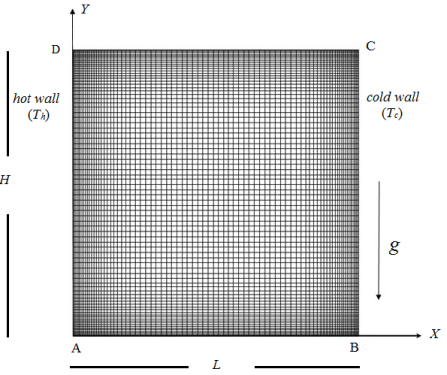Figure 1. Schematic diagram of 2D cavity

2. Governing Equations

The dimensionless form for the continuity, momentum and energy equations with inclusion of the buoyant Boussinesq approximations for the density variation are written as

$\nabla \cdot \boldsymbol{V}=0$   (1)

$\boldsymbol{V} \cdot \nabla \boldsymbol{V}=-\nabla P+\operatorname{Pr} \nabla^{2} \boldsymbol{V}+\operatorname{Ra} \operatorname{Pr} T^{*}$     (2)

$\boldsymbol{V} \cdot \nabla T^{*}=\nabla^{2} T^{*}$     (3)

In the above Eqns. $(1)-(3), \boldsymbol{V}=(U, V), P, T^{*}$ represent the dimensionless velocity vector along the $(X, Y)$ directions, pressure and temperature respectively. The following non-dimensional parameters were used. $X=\frac{x}{L}, Y=\frac{y}{L}, U=\frac{u L}{\alpha}, V=\frac{v L}{\alpha}, P=\frac{p L^{2}}{\rho \alpha^{2}}, T^{*}=\frac{T-T_{C}}{\Delta T}, P r=\frac{v}{\alpha}$ and $R a=\frac{g \beta(\Delta T) L^{3}}{v \alpha},$ where the notations are explained in the Nomenclature. $\Delta T$ be the temperature difference between two vertical walls, $\Delta T=\left(T_{H}-T_{C}\right) .$

V= 0 at X = 0, 1; Y = 0, 1.

$T^{*}=1$ at $X=0,$ and $T^{*}=0$ at $X=1$ and $\frac{\partial T^{*}}{\partial Y}=0,$ at $Y=0,1$    (4)

The non-dimensional heat transfer rate at the hot wall is calculated by the Nusselt number whose local value along the hot wall is given by $N u=\left(\frac{\partial T^{\prime \prime}}{\partial X}\right)_{X=0} \cdot$ The average Nusselt number $\overline{N u}$ is obtained by integrating the local Nusselt number along the hot wall and is calculated as $\overline{N u}=-\int_{X=0}^{X=1}\left(\frac{\partial T^{*}}{\partial X}\right) d X$.

3. Numerical Method and Validation

The finite volume method  is employed to discretize the Eqns. (1-3). In this method, the computational domain of the cavity is divided into rectangular volumes with velocity vector calculated at the corner of the volume and the scalar unknowns P and T*are positioned in the center of the finite volume. Established open source CFD package OpenFOAM, has been utilized. The geometry, volume and boundary conditions are set in the buoyantBoussinesqSimpleFoam. The upwind numerical method of second order was employed for the derivatives and the CGS method to accelerate convergence. The QUICK and Gauss linear schemes used to discretize the divergent and Laplacian terms respectively. The post processing is done in Tecplot, the Paraview and LibreOffice Calc.

Table 1. Validation of 2D results in terms of $\overline{N u}(H / L=1)$

 Ra 103 104 105 106 Grid size 80x80 80x80 80x80 160x160 De Vahl Davis and Jones  1.1182 2.243 4.519 8.799 Markatos and Pericleous  1.108 2.201 4.430 8.754 Fusegi et al.  1.105 2.302 4.646 9.012 Barakos et al.  1.114 2.245 4.510 8.806 Rincon Casado et al.  1.118 2.241 4.522 8.819 Present Work 1.128 2.194 4.515 8.843

## f2a.png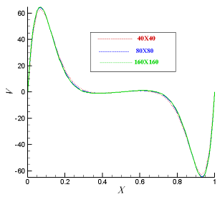(a)

## f2b.png(b)
Figure 2. Grid independent test for Ra = 105. (a) Profiles of velocity (V) component at mid height of cavity (b) Temperature (T*) distribution at mid height of cavity

The Table 1 tabulates the comparison of the simulated results obtained by different authors in the study of a square cavity (AR = 1) with lateral walls at different temperatures. The Ra is varied through the cavity size and the variable of interest is the $\overline{N u}$.  From the Table 1, it can be observed that $\overline{N u}$ obtained in the present study is in good agreement with those in the literature.

Figure 2 shows the V and T* solutions calculated using different meshes, namely 402, 802 and 1602 for Ra = 105. Figures 2 (a) and (b) show profiles of velocity component and temperature distribution at mid height of the cavity, respectively. Also it is observed that currently employed 802 uniformly stretched mesh results did not deviate much from those when the mesh resolution was amplified by 50 %. Thus the 802 mesh resolution was employed in the present study.

4. Results and Discussion

In this section, the concept of Streamlines, isotherms, energy streamlines and field synergy principle are explained and used in the present study to visualize and analyse the flow behavior.

4.1 Streamlines

Figure 3 demonstrates the simulated streamlines for various values of Ra and AR. As anticipated due to uniformly heated vertical walls in which the flow along the hot left wall and the flow down along the cold right wall developing a roll inside the cavity with clockwise rotation. It is observed, when Ra is 103, principal vortex seems to be main characteristic of the flow. As Ra increases, stream function value also increases, i.e., the flow rate increases. Due to convection, secondary circulation formed at the left corner of the top wall and also the hot fluid shift towards the left corner of the cavity. Also it is noticed that vortex happen to be become elliptic and split into two or more. The rotation is clockwise owing to a very small positive temperature gradient at the centre of the cavity. The vortices become narrow for even high Ra improving the stratification of the flow at the principal part of the cavity.

Figures 3 (a, d, g, j) show the streamlines in enclosures of buildings for Ra: 103-106 and AR= 0.5. In Fig. 3(a), the innermost vortex seems to be most important feature of the flow due to dominant conduction mode of heat transfer. As Ra increases, the innermost vortex breaks up into two vortices noticed in Figure 3(d). The vortices move close to the walls, providing a space for third vortex to generate in the direction of horizontal walls as shown in Figure 3(g). As Ra further increases to 106, the convection mechanism becomes more evident and the velocity at the boundaries has higher value than velocity at the center of the cavity because the fluid is moving very fast at the boundaries. Hence there is formation of two more vortices at the top left corner and bottom right corner of the cavity as observed in Figure 3(j). Figures 3(b, e, h, k) show the simulated results for Ra: 103-106 and AR = 1. For lower value of Ra = 103, the innermost vortex seems to be dominant feature of the flow (Figure 3(b)) and as Ra increases to 104, the vortex attains an elliptic shape (Figure 3(e)). For further increasing values of Ra, as shown in Figure 3(h), the innermost vortex breaks up into two vortices horizontally. As Ra still increases to 106, the innermost vortex breaks up into two vortices noticed in Figure 3(k). Figures 3(c, f, i, l) streamline flow for Ra: 103-106 numbers and AR = 2. In Figure 3(c, f), the primary vortex seems to be dominant feature of the flow. As Ra increases to 105, it is noticed that primary vortex breaks up into two vortices in the vertical direction as observed in Figure 3(i). As 105<Ra ≤ 106 and especially when equal to 106, it is observed that the third vortex formed at the center of the cavity along with two more emerging eddies formed at corners of top left and bottom right walls. This is due to the fact that the velocity at the boundaries is higher than velocity at the center of the cavity in Figure 3(l). [25, 35-37].

## f3a.png(a)

## f3b.png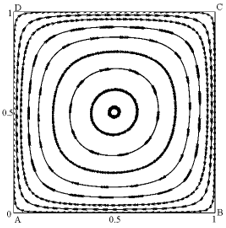(b)

## f3c.png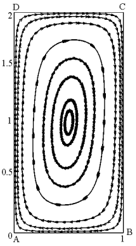(c)

## f3d.png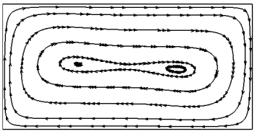(d)

## f3e.png(e)

## f3f.png(f)

## f3g.png(g)

## f3h.png(h)

## f3i.png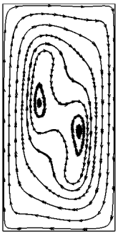(i)

## f3j.png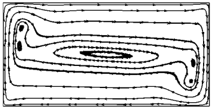(j)

## f3k.png(k)

## f3l.png(l)

Figure 3. The simulated streamlines for Ra = 103 (a, b, c), 104 (d, e, f) , 105 (g, h, i) and 106 (j, k, l) for different AR = 0.5 (a, d, g, j),  1 (b, e, h, k) and 2(c, f, i, l)

4.2 Isotherms

Figure 4 depicts the simulated isotherms for different values of Ra and AR. When Ra = 103, the following heat transfer modes are comparable i.e., conduction and convection. The temperature distribution and flow fields are affected from the above two modes. When Ra increases moderately, weaker secondary recirculating zone is formed over the top of the cavity. The primary recirculation cell fills the entire space and becoming larger towards the secondary recirculating cell formed at the top. In this case, the prominent point is that there exists a bigger isothermal section on the upper half of enclosure.

For low Ra (= 103) and as AR increases from 0.5 to 2, it is observed the presence of isotherms which are almost vertical as displayed in Figures 4(a, b, c). This is due to conduction of heat, which is transferred between hot wall and cold wall. When the mode of heat transport changes from conduction to convection, the isotherms departs from the vertical to curved position as depicted in Figures 3(d - i). After this transition as noticed in Figures. 3(j, k, l) there is an appearance of horizontal isotherms at the center of the cavity and vertical isotherms at the boundaries. Similar vertical structures are noticed by de Vahl Davis and Jones and Markatos and Pericleous, Rincon-Casado et al. [25, 36, 37].

## f4a.png(a)

## f4b.png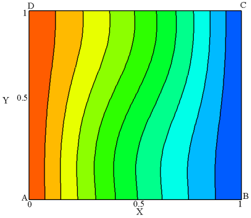(b)

## f4c.png(c)

## f4d.png(d)

## f4e.png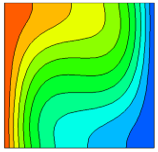(e)

## f4f.png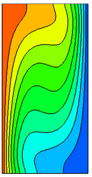(f)

## f4g.png(g)

## f4h.png(h)

## f4i.png(i)

## f4j.png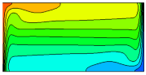(j)

## f4k.png(k)

Figure 4. The simulated isotherms for Ra=103(a, b, c), 104(d, e, f), 105(g, h, i) and 106 (j, k, l) for different AR=0.5(a, d, g, j), 1(b, e, h, k), and 2(c, f, i, l) respectively

4.3 Energy streamlines

In a 2D flow, the energy streamlines are obtained by solving a Poisson equation of the type: $\nabla^{2} \Phi=(\nabla \times \overline{E}) \cdot \overline{k},$ where $\Phi$ is called the energy streamfunction and $\overline{k}$ is the unit vector. For 2D convective heat transfer system, in Cartesian coordinate system, $\overline{E}$ can be written as

$\overline{E}=\rho \boldsymbol{V}\left(\frac{1}{2} \boldsymbol{V}^{2}+h\right)-\boldsymbol{V} \cdot \overline{\sigma}-K \nabla T$    (5)

where the notations are explained in the Nomenclature. Energy streamlines include contribution of energy due to surface forces and energy fluxes. Hence, they can provide a complete view for configurations where these impacts are significant. Therefore, the simultaneous utilization of energy streamlines and heatlines are helpful to examine the quantitative details regarding the participation of the extra energy fluxes from the energy stream function. The energy flux density vectors are utilized to reveal an imaginary energy flow path, which is like pathline trace . According to Mahmud and Fraser , 'free energy streamline' is defined as streamline of the flow that originates at the hot wall, forms circular rolls and then intersects at the cold wall. This line is due to the participation of convective thermal energy and kinetic energy is negligible in comparison to conductive thermal energy, where the velocity components are modest. While 'trapped energy streamline' is streamline of the flow that begins at hot wall, moves through the fluid and creates a closed loop because of kinetic energy and convective thermal energy dominates the conductive thermal energy.

Figures 5(a, d, g, j) demonstrates streamline flow in the cavity for Ra: 103 - 106 and with fixed AR = 0.5. In Figure 5(a), it is noted the existence of free horizontal energy streamlines at Ra = 103, i.e., the flow started at left hot wall, moves through the fluid and then terminated at the right cold wall and trapped streamlines formed at the center of the cavity. It is observed that trapped energy streamlines occupy more space when Ra increases to 105 as noticed in Figures 5(d, g). When Ra = 106, single eddy emerged at the left center of the cavity (Figure 5(j)). Similar pattern is also observed in Figures. 5(b, e, h, k) when AR = 1. When Ra is small vertical energy streamlines formed (Figure 5(b)) and as Ra increases the free and trapped energy streamlines are observed at the left boundary and at the center, respectively. When Ra = 106, it is observed the two emerging eddies each at the top left and top right corners of the cavity are also similar to the trapped energy streamlines and occupy more region in the cavity (Figure 5(k)).

## f5a.png(a)

## f5b.png(b)

## f5c.png(c)

## f5d.png(d)

## f5e.png(e)

## f3f.png(f)

## f5g.png(g)

## f5h.png(h)

## f3i.png(i)

## f3j.png(j)

## f3k.png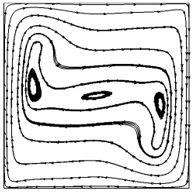(k)

## f3l.png(l)

Figure 5. The simulated energy streamlines for Ra=103(a, b, c), 104(d, e, f), 105(g, h, i) and 106 (j, k, l) for different AR=0.5(a, d, g, j), 1(b, e, h, k) and 2(c, f, i, l) respectively.

Figures 5(c, f, i, l) show flow inside the cavity with AR=2. When Ra =104, the free energy streamlines at the left boundary are noticed while the trapped energy streamlines at the center appear in the vertical direction and occupy more region in the cavity. When Ra increased further more (=106) circularly trapped streamlines in vertical direction are observed (Figure 5(i)) along with one emerging eddy at left top of cavity (Figure 5(l)) .

4.4 Field synergy angle

Local intersection angle is given by (Guo et al. (1998))

$\beta_{m}=\cos ^{-1}\left(\frac{V \cdot \nabla T}{|V||\nabla T|}\right)$    (6)

The value of $\beta$ is the angle between the velocity vector and heat flow vector (temperature gradient). In Eq. $(6),$ there are three scalar fields, namely, $|\boldsymbol{V}|,|\nabla T|$ and $\operatorname{Cos} \beta_{m}$ or two $\mathrm{or}$ two $\mathrm{er}$ vector fields, namely $\boldsymbol{V}$ and $\nabla T .$ Thus, along with the velocity vector and the temperature gradient, their synergy determines the strength of the convective heat transfer.

Figure 6 shows the contours of $\beta_{m}$ value for different values of $R a$ and $A R .$ When $A R=0.5,$ at the boundaries the synergy angle decreases with the increase of $R a(\text { Figures } 6(a, d, g, j))$ Similar pattern is observed when $A R=1$ and $2 .$ It is also observed that the flow is symmetric and the included angle has lower values in the bottom half of the cavity in-comparison with the upper half of the cavity. This implies that the velocity and temperature gradient vectors aligned parallel and perpendicular at the upper half and lower half of the cavity, respectively. This is due to the dominant heat transfer mechanism that switches from conduction to convection state. More conduction/convection occurs in the upper/lower half of the cavity. The interchange of these two states is not symmetric with increasing Ra. Thus, there is a reduction of the intersection angle between velocity and temperature gradient in the lower half of the cavity and thereby in this region there is an effective enhancement of convective heat transfer.  It can be said that the synergy is improved at the locations where the isotherms crossly intersected by the flow velocities.

## f6a.png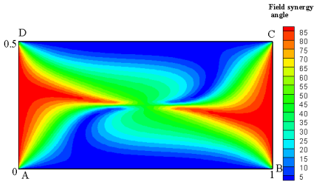(a)

## f6b.png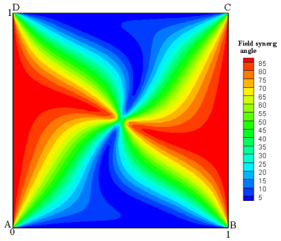(b)

## f6c.png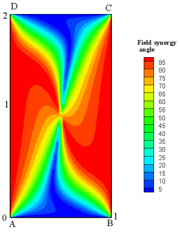(c)

## f6d.png(d)

## f6e.png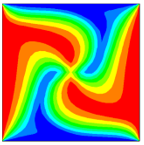(e)

## f6f.png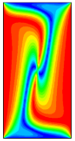(f)

## f6g.png(g)

## f6h.png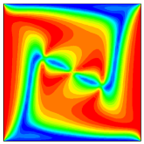(h)

## f6i.png(i)

## f6j.png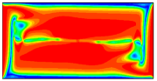(j)

## f6k.png(k)

## f6l.png(l)

Figure 6. The simulated synergy angle for Ra=103(a, b, c), 104(d, e, f), 105(g, h, i) and 106 (j, k, l) for different AR=0.5(a, d, g, j), 1(b, e, h, k) and 2(c, f, i, l) respectively

Table 2. Average synergy angle βmvariation with Ra under different AR

 Ra βm AR = 0.5 AR = 1 AR = 2 103 74.25 76.99 80.21 104 77.12 79.51 81.58 105 78.58 80.82 81.68 106 80.21 81.32 81.75

Table 2 shows the variation of average synergy angle with Ra under different values of AR. Field synergy principle could disclose the character of improved heat transport. From the tabulated values, it can be seen that with the increase of Ra (103 ~ 106) and for a fixed AR (0.5-2), βm increases. It is noted that as Ra increases, synergy angle increases for different AR. This result implies that synergy between temperature field and velocity gets worse, which leads to the mild growth rate of $\overline{N u}$. The synergy angle of buoyant-aiding flow is greater while the buoyant-opposing flow is significantly less than that of natural convection flow when AR = 1. This result is leads to the fact that the relation between temperature field and velocity at buoyant-aiding flow is at the worst situation, and leads to the increasing values of $\overline{N u}$ .
5. Conclusion

The present paper demonstrates the energy pathlines and field synergy principle to visualize the natural convective flow and thermal characteristics for laminar regime (103Ra ≤106) in enclosures with aspect ratio (AR) of 0.5, 1 and 2. For the small values Ra and AR=0.5 the innermost vortex seems to be dominant feature of the flow. As Ra increases, the innermost vortex breaks up into two vortices thus providing a space for third vortex to emerge in the horizontal direction. As Ra further increases to 106, the velocity at the boundaries is higher than velocity at the center of the cavity because of the increased convection. Hence formation of two more vortices at the top left corner and bottom right corner of the cavity is observed. Similar pattern is observed in the vertical direction when AR=2.

When Ra=103 and AR increases from 0.5 to 2, almost vertical isotherms are observed due to the initial conduction state. When the mode of heat transport changes from conduction to convection the isotherms depart from the vertical position, become curved and then appear as the horizontal isotherms at the center of the cavity and vertical isotherms at the boundaries.

For small values of Ra, the horizontal free energy streamlines and trapped energy streamlines are observed. As Ra increases, the trapped energy streamlines occupy more space at the center of the cavity. When AR=0.5, single eddy at left center of the cavity is formed, whereas when AR=2, two emerging eddies each at the top left and top right corners of the cavity are observed.

When Ra increases, synergy angle decreases for different AR. This result implies that synergy between temperature field and velocity gets worse, which leads to the mild growth rate of $\overline{N u}$ The synergy angle of buoyant-aiding flow is greater while the buoyant-opposing flow is significantly less than that of natural convection flow when AR=1. This result is leads to the fact that the relation between temperature field and velocity at buoyant-aiding flow is at the worst situation, and leads to the increasing values of $\overline{N u}$

Nomenclature
 AR aspect ratio (H/L) Cp Specific heat $\overline{E}$ energy flux density vector, W m-2 g gravitational acceleration m s-2 Gr Grashof number ($=\frac{g \beta(\Delta T) L^{3}}{v^{3}}$) H height of the cavity, m K Thermal conductivity, W m-1 K-1 L Length of the cavity, m Nu Local Nusselt number $\overline{\mathrm{Nu}}$ Average Nusselt number p non dimensional pressure Pr Prandtl number ($=\frac{v}{\alpha}$) Ra Rayleigh number $=\frac{g \beta(\Delta T) L^{3} P r}{v^{3}}$ Re Reynolds number ($=\mathrm{U}_{0} \mathrm{h} / \mathrm{v}$) Ri Richardson number (= Gr.Re-2) T Dimensional temperature, K T* Dimensionaless temperature $=\frac{\mathrm{T}-\mathrm{T}_{\mathrm{C}}}{\Delta \mathrm{T}}$ U0 Top wall velocity, m s-1 V Dimensionless velocity vector X,Y Dimensionless distance along x and y coordinates Greek letters α coefficient of thermal diffusion, m2 s-1 β coefficient of thermal expansion, K-1 v molecular kinematic viscosity, m2 s-1 σ viscous stress tensor, N m-2 ρ Density, kg m-3 $\beta_{\mathrm{m}}$ field synergy angle between V and the temperature gradient $\Delta \mathrm{T}$ characteristic temperature difference $\nabla \mathrm{T}^{*}$ dimensionless temperature
References

 Altac, Z., Uğurlubilek, N. (2016). Assessment of turbulence models in natural convection from two and three dimensional rectangular enclosures. International Journal of Thermal Sciences, 107: 237-246. http://dx.doi.org/10.1016/j.ijthermalsci.2016.04.016

 Obyn, S., Van Moeseke, G. (2015). Variability and impact of internal surfaces convective heat transfer coefficients in the thermal evaluation of ice buildings. Applied Thermal Engineering, 87: 258-272. https://doi.org/10.1016/j.applthermaleng.2015.05.030

 Bejan, A. (2004). Convection heat transfer. John Wiley & Sons, New York. http://dx.doi.org/10.1002/9781118671627

 Awbi, H., Hatton, A. (1999). Natural convection from heated room surfaces. Energy and Buildings, 30: 233-244. http://dx.doi.org/10.1016/S0378-7788(99)00004-3

 Novoselac, A., Burley, B., Srebric, J. (2006). Development of new and validation of existing convection correlations for rooms with displacement ventilation systems. Energy and Buildings, 38: 163-173. http://dx.doi.org/10.1016/j.enbuild.2005.04.005

 Fusegi, T., Hyun, J.M., Kuwahara, K., Farouk, B. (1991). A numerical study of three dimensional natural convection in a differentially heated cubical enclosure. International Journal of Heat and Mass Transfer, 34: 1543-1557. http://dx.doi.org/10.1016/0017-9310(91)90295-P

 Tric, E., Labrosse, G., Betrouni, M. (2000). A first incursion into the 3D structure of natural convection of air in a differentially heated cubic cavity, from accurate numerical solutions. International Journal of Heat and Mass Transfer, 43: 4043-4056. http://dx.doi.org/10.1016/S0017-9310(00)00037-5

 Wakashima, S., Saitoh, T.S. (2004). Benchmark solutions for natural convection in a cubic cavity using the high-order time–space method. International Journal of Heat and Mass Transfer, 47: 853-864. http://dx.doi.org/10.1016/j.ijheatmasstransfer.2003.08.008

 Kimura, S., Bejan, A. (1983). The Heatline visualization of convective heat transfer. Journal of Heat Transfer, 105: 916-919. http://dx.doi.org/10.1115/1.3245684

 Costa, V. (1999). Unification of the streamline, heatline and massline methods for the visualization of two-dimensional transport phenomena. International Journal of Heat and Mass Transfer, 42: 27-33. http://dx.doi.org/10.1016/S0017-9310(98)00138-0

 Costa, V. (2003). Unified streamline, heatline and massline methods for the visualization of two-dimensional heat and mass transfer in anisotropic media. International Journal of Heat and Mass Transfer, 46: 1309-1320. http://dx.doi.org/10.1016/S0017-9310(02)00404-0

 Mahmud, S, Fraser, R. (2007). Visualizing energy flows through energy streamlines and pathlines. International Journal of Heat and Mass Transfer, 50: 3990-4002. http://dx.doi.org/10.1016/j.ijheatmasstransfer.2007.01.032

 Hooman, K. (2010). Energy flux vectors as a new tool for convection visualization. International Journal of Numerical Methods for Heat & Fluid Flow, 20: 240-249. http://dx.doi.org/10.1108/09615531011016984

 Bararnia, H., Hooman, K., Ganji, D. (2011). Natural convection in a nanofluids-filled portioned cavity: The lattice-Boltzmann method. Numerical Heat Transfer, Part A: Applications, 59: 487-502. http://dx.doi.org/10.1080/10407782.2011.541195

 Malik, S., Nayak, A. (2017). MHD convection and entropy generation of nanofluid in a porous enclosure with sinusoidal heating. International Journal of Heat and Mass Transfer, 111: 329-345. http://dx.doi.org/10.1016/j.ijheatmasstransfer.2017.03.123

 Guo, G., Li, D., Wang, B. (1998). A novel concept for convective heat transfer enhancement. International Journal of Heat and Mass Transfer, 41: 2221-2225. http://dx.doi.org/10.1016/S0017-9310(97)00272-X

 Guo, Z., Tao, W., Shah, R. (2005). The field synergy (coordination) principle and its applications in enhancing single phase convective heat transfer. International Journal of Heat and Mass Transfer, 48: 1797-1807. http://dx.doi.org/10.1016/j.ijheatmasstransfer.2004.11.007

 Mallinson, G.D., De Vahl Davis, G. (1977). Three dimensional natural convection in a box: A numerical study. Journal of Fluid Mechanics, 83: 1-31. http://dx.doi.org/10.1017/S0022112077001013

 Pepper, D.W., Hollands, K.G.T. (2002). Summary of benchmark numerical studies for 3D natural convection in an air-filled enclosure. Numerical Heat Transfer, Part A: Applications, 42: 1-11. https://doi.org/10.1080/10407780290059396

 Ravnik, J., Skerget, L., Zunic, Z. (2008). Velocity–vorticity formulation for 3D natural convection in an inclined enclosure by BEM. International Journal of Heat and Mass Transfer, 51: 4517-4527. http://dx.doi.org/10.1016/j.ijheatmasstransfer.2008.01.018

 Rincon-Casado, A., Sanchez de la Flor, F.J., Chacon Vera, E., Sanchez Ramos, J. (2017) New natural convection heat transfer correlations in enclosures for building performance simulation. Engineering Applications of Computational Fluid Mechanics, 11(1): 340-356. https://doi.org/10.1080/19942060.2017.1300107

 Catton, I. (1988). Natural convection in enclosures. Journal of Heat Transfer, 110(4b). http://dx.doi.org/10.1115/1.3250619

 Ostrach, S. (1972). Natural convection in enclosures. Advances in Heat Transfer, 8: 161-227. http://dx.doi.org/10.1016/S0065-2717(08)70039-X

 Leong, W.H., Hollands, K.G.T., Brunger, A.P. (1998). On a physically-realizable benchmark problem in internal natural convection. International Journal of Heat and Mass Transfer, 41: 3817-3828. http://dx.doi.org/10.1016/S0017-9310(98)00095-7

 Betts, P.L., Bokhari, I.H. (2000). Experiments on turbulent natural convection in an enclosed tall cavity. International Journal of Heat and Fluid Flow, 21: 675-683. http://dx.doi.org/10.1016/S0142-727X(00)00033-3

 Mamun, M.A.H., Leong, W.H., Hollands, K.G.T., Johnson, A. (2003). Cubical-cavity natural-convection benchmark experiments: An extension. International Journal of Heat and Mass Transfer, 46: 3655–3660. http://dx.doi.org/10.1016/S0017-9310(03)00155-8

 Ampofo, F. (2005). Turbulent natural convection of air in a non partitioned or partitioned cavity with differentially heated vertical and conducting horizontal walls. Experimental Thermal and Fluid Science, 29: 137-157. http://dx.doi.org/10.1016/j.expthermflusci.2004.02.005

 Bairi, A. (2008). Nusselt-Rayleigh correlations for design of industrial elements: Experimental and numerical investigation of natural convection in tilted square air filled enclosures. Energy Conversion and Management, 49: 771-782. http://dx.doi.org/10.1016/j.enconman.2007.07.030

 Saury, D., Rouger, N., Djanna, F., Penot, F. (2011). Natural convection in an air-filled cavity: Experimental results at large Rayleigh numbers. International Communications in Heat and Mass Transfer, 38: 679-687. http://dx.doi.org/10.1016/j.icheatmasstransfer.2011.03.019

 Inan, T., Başaran, T., Ezan, M.A. (2016). Experimental and numerical investigation of natural convection in a double skin facade. Applied Thermal Engineering, 106: 1225-1235. http://dx.doi.org/10.1016/j.applthermaleng.2016.06.124

 Barakos, G., Mitsoulis, E., Assimacopoulos, D. (1994). Natural convection flow in a square cavity revisited: Laminar and turbulent models with wall functions. International Journal for Numerical Methods in Fluids, 18: 695-719. http://dx.doi.org/10.1002/fld.1650180705

 Markatos, N.C., Pericleous, K.A. (1984). Laminar and turbulent natural convection in an enclosed cavity. International Journal of Heat and Mass Transfer, 27: 755-772. http://dx.doi.org/10.1016/0017-9310(84)90145-5

 De Vahl Davis, G., Jones, I.P. (1983). Natural convection in a square cavity: A comparison exercise. International Journal for Numerical Methods in Fluids, 3: 227-248. http://dx.doi.org/10.1002/fld.1650030304

 Lari, K., Baneshi, M., Gandjalikhan Nassab, S.A., Komiya, A., Maruyama, S. (2011). Combined heat transfer of radiation and natural convection in a square cavity containing participating gases. International Journal of Heat and Mass Transfer, 54: 5087-5099. http://dx.doi.org/10.1016/j.ijheatmasstransfer.2011.07.026

 Osman, T., Poole, R.J., Chakraborty, N. (2012). Influences of boundary conditions on laminar natural convection in rectangular enclosures with differentially heated side walls. International Journal of Heat and Fluid Flow, 33: 131-146. https://doi.org/10.1016/j.ijheatfluidflow.2011.10.009

 Trias, F.X., Gorobets, A., Soria, M., Oliva, A. (2010). Direct numerical simulation of a differentially heated caviity of aspect ratio 4 with Rayleigh numbers up to 1011 – Part I: Numerical methods and time-averaged flow. Inter-national Journal of Heat and Mass Transfer, 53: 665-673. http://dx.doi.org/10.1016/j.ijheatmasstransfer.2009.10.026

 Patankar, S.V., Spalding, D.B. (1972). A calculation procedure for heat, mass and momentum transfer in three dimensional parabolic flows. International Journal of Heat and Mass Transfer, 15: 1787–1806. http://dx.doi.org/10.1016/0017-9310(72)90054-3

 Cheng, Y.P., Lee, T.S., Low, H.T. (2006). Numerical analysis of mixed convection in three dimensional rectangular channel with flush-mounted heat sources based on field synergy principle. International Journal for Numerical Methods in Fluids, 52: 987-1003. http://dx.doi.org/10.1002/fld.1218